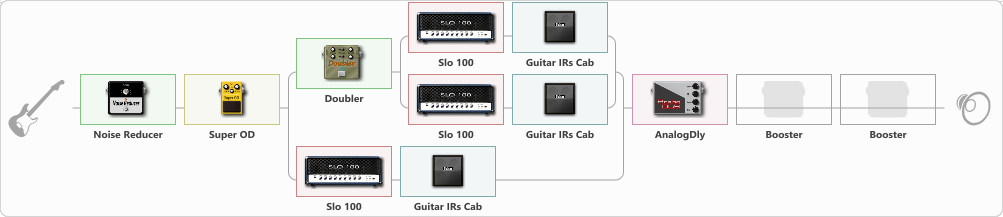Discussion in 'ToneLib-GFX presets' started by STasER009, May 6, 2023.

humbucker

Effects chain:Effect: "Noise Reducer" (Dynamics / Filter), active - "yes"
{
"Sens" = 100
"Mode" = Soft
}

Effect: "Super OD" (Overdrive / Distortion), active - "yes"
{
"Drive" = 8
"Tone" = 80
"Level" = 91
}

Effect: "Splitter" (Dynamics / Filter)
{
"A-Bypass" = Off
"A-Pan" = -25
"A-Level" = 55
"B-Bypass" = Off
"B-Pan" = 25
"B-Level" = 55
"Width" = 0

'A' branch:
{

Effect: "Doubler" (Dynamics / Filter), active - "yes"
{
"A-Dly" = 75
"A-Inv" = Off
"A-Level" = 75
"B-Dly" = 50
"B-Inv" = Off
"B-Level" = 50
}

Effect: "Splitter" (Dynamics / Filter)
{
"A-Bypass" = Off
"A-Pan" = -25
"A-Level" = 55
"B-Bypass" = Off
"B-Pan" = 25
"B-Level" = 55
"Width" = 0

'A' branch:
{

Effect: "Slo 100" (Amp simulators), active - "yes"
{
"Gain" = 50
"Bass" = 50
"Middle" = 60
"Treble" = 60
"Presence" = 80
"Master" = 50
"Level (dB)" = 0
}

Effect: "Guitar IRs Cab" (Cabinets), active - "yes"
{
"Model" = Engl Pro (4x12")
"Mic Position" = Middle
"Mic Distance" = Far
"Low Cut (Hz)" = 0
"Hi Cut (kHz)" = 16.0
"Mix" = 100
"Level (dB)" = 10
}
}
'B' branch:
{

Effect: "Slo 100" (Amp simulators), active - "yes"
{
"Gain" = 50
"Bass" = 50
"Middle" = 60
"Treble" = 60
"Presence" = 80
"Master" = 50
"Level (dB)" = 0
}

Effect: "Guitar IRs Cab" (Cabinets), active - "yes"
{
"Model" = Engl Pro (4x12")
"Mic Position" = Middle
"Mic Distance" = Middle
"Low Cut (Hz)" = 0
"Hi Cut (kHz)" = 20.0
"Mix" = 100
"Level (dB)" = 10
}
}
}
}
'B' branch:
{

Effect: "Slo 100" (Amp simulators), active - "yes"
{
"Gain" = 50
"Bass" = 50
"Middle" = 60
"Treble" = 60
"Presence" = 80
"Master" = 50
"Level (dB)" = 0
}

Effect: "Guitar IRs Cab" (Cabinets), active - "yes"
{
"Model" = Engl Pro (4x12")
"Mic Position" = Middle
"Mic Distance" = Far
"Low Cut (Hz)" = 0
"Hi Cut (kHz)" = 16.0
"Mix" = 100
"Level (dB)" = 10
}
}
}

Effect: "AnalogDly" (Delay), active - "yes"
{
"Time" = 44
"Feedback" = 24
"Tone" = 65
"Mix" = 65
}

Effect: "Booster" (Dynamics / Filter), active - "no"
{
"Gain" = 100
}

Effect: "Booster" (Dynamics / Filter), active - "no"
{
"Gain" = 100
}

Note: You will need to download and install the ToneLib-GFX software to use the preset.